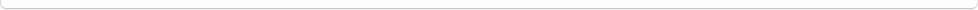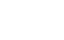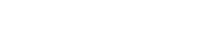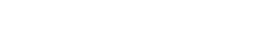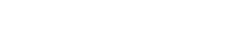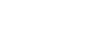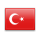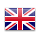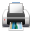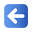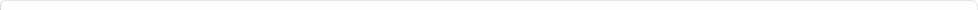Description of Individual Course Units
 Course Unit Code Course Unit Title Type of Course Unit Year of Study Semester Number of ECTS Credits 2111001103 Compulsory 1 1 4
Level of Course Unit
Short Cycle
Objectives of the Course
"With this course student should gain commercial knowledge and skills related to mathematical calculation procedures. "
Name of Lecturer(s)
ÖĞR.GÖR.DİNÇER ATASOY
Learning Outcomes
 1 Ratio and average calculations can be done. 2 Ratio and average calculations can be done. 3 percentage calculations can be done. 4 percentage calculations can be done. 5 Cost, profit and loss calculations can be done. 6 Cost, profit and loss calculations can be done. 7 Mix designs are done 8 Mix designs are done 9 Interest calculations 10 Interest calculations 11 Discount calculations. 12 Discount calculations.
Mode of Delivery
Daytime Class
Prerequisites and co-requisities
None
Recommended Optional Programme Components
None
Course Contents
"Ratio and proportion, average, percentage and per thousand accounts, Profit or Loss percentage calculation, the cumulative and average profit calculations, cost and sales accounts, mix accounts, simple interest (interest amount, capital, interest rate, term-term, the accumulated amount calculation of the interest, antisipe interest, the domestic interest rate, foreign interest rates, average interest rates, commercial and real interest rate calculation), Compound Interest (Future value, present value, the nominal interest rate, effective interest, proportionate to the interest percentage rate, the equivalent interest rate, compound discount, equivalent circuit payments, the calculation of payments per circuit), the discount, annuities
Weekly Detailed Course Contents
 Week Theoretical Practice Laboratory 1 "Ratio and proportion " 2 "The correct proportion, inverse proportion, Compound Proportion " 3 "Average " 4 "Percent and per thousand accounts " 5 "Calculation of Internal and External Percentage " 6 "Cost and sales accounts " 7 "Average profit, the cumulative profit. " 8 Alloy and mixture calculations. 9 "Simple interest calculations " 10 "Compound interest calculations " 11 Foreign Discount Method, Internal Discount Method 12 "Equivalent, merged, and the equivalent bonds " 13 annuities 14 annuities
"Ticari matematik (Lec.. Dinçer Atasoy nobel yayınları) , Other resources and documents.
Planned Learning Activities and Teaching Methods
Assessment Methods and Criteria
 Term (or Year) Learning Activities Quantity Weight Midterm Examination 1 100 SUM 100 End Of Term (or Year) Learning Activities Quantity Weight Final Examination 1 100 SUM 100 Term (or Year) Learning Activities 40 End Of Term (or Year) Learning Activities 60 SUM 100
Language of Instruction
Turkish
Work Placement(s)
None